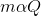The mass of the substance (m) deposited or liberated at an electrode is directly proportional to the quantity of electricity or charge (Q) passed.

In the mathematical form, this law can be represented as follows:Removing the proportionality sign gives m = ZQ, where m is the mass in grams (g), Q is measured in Coulombs (C), and Z is the proportionality constant in g/C (in grams per coulomb) and is also known as the electrochemical equivalent, which is the mass of a substance produced at the electrode during electrolysis by one Coulomb of charge.

Faraday further observed that 1 Faraday (96,485C) of charge liberates 1 gram equivalent of the substance at the electrodes.

This means that 1C will liberate one gram equivalent of a substance/96,485, which is the electrochemical equivalent (Z) of the substance.

This goes to a relationship between electrochemical equivalent (Z) and equivalent weight or equivalent mass (E) of a substance, and this can be expressed as:

Z = Equivalent weight/96,485, or Z = E/96,485

Moving back to the equation m = ZQ, it can alternately be written as:

m = Z x I x t (since Q = I x t)

m = E x I x t /96,485 (since Z = E/96,485)